Welcome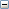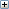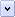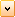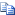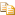SetEquationAndConfigurationOption Method (IEquationMgr)

Modifies the equation at the specified index for the specified configurations.

# .NET Syntax

Visual Basic (Declaration)
```Function SetEquationAndConfigurationOption( _
ByVal Index As System.Integer, _
ByVal Equation As System.String, _
ByVal WhichConfigurations As System.Integer, _
ByVal ConfigNames As System.Object _
) As System.Integer```
Visual Basic (Usage)
```Dim instance As IEquationMgr
Dim Index As System.Integer
Dim Equation As System.String
Dim WhichConfigurations As System.Integer
Dim ConfigNames As System.Object
Dim value As System.Integer

value = instance.SetEquationAndConfigurationOption(Index, Equation, WhichConfigurations, ConfigNames)```
C#
```System.int SetEquationAndConfigurationOption(
System.int Index,
System.string Equation,
System.int WhichConfigurations,
System.object ConfigNames
)```
C++/CLI
```System.int SetEquationAndConfigurationOption(
&   System.int Index,
&   System.String^ Equation,
&   System.int WhichConfigurations,
&   System.Object^ ConfigNames
) ```

#### Parameters

Index
0-based index of the equation to modify
Equation
String containing the modified equation (see Remarks)
WhichConfigurations
Configuration option as defined in swInConfigurationOpts_e
ConfigNames
Array of the names of the configurations to which to add this equation; valid only if WhichConfigurations is set to swInConfigurationOpts_e.swSpecifyConfiguration, in which case, include the name of the current configuration in this array

#### Return Value

Index of equation if successfully modified, -1 if error

# Remarks

To add an equation using the SOLIDWORKS user interface, you must embed the names of dimensions and global variables in double quotes:

• Global variable assignment:
"B" = 2
• Component equation:
"N_SPOKES@CirPattern" = "BARLENGTH@Sketch2" /10
• Dimension equation that uses the Visual Basic IIf function:
"D1@Extrude2" = (IIf("D1@Extrude3">20, 15, 6))+5
• Dimension equation that sets a dimension to the current value:
"D1@Extrude2" =
• Dimension equation that modifies the right-hand side of an already existing dimension equation:
"D1@Extrude2" = 0.05

NOTES:

• To modify an equation:
• you must specify Equation with the names of dimensions and global variables in double double quotes and the entire equation in double quotes. The examples for IEquationMgr::Add3 show how to do this.Global variables cannot be set to current values using this method.
• added directly to an assembly component's model, you must call IAssemblyDoc::EditPart2 before calling this method.
• If you change the active configuration, then you must call IModelDoc2::GetEquationMgr again.
• If the model has just one configuration, then use IEquationMgr::Equation.

# Availability

SOLIDWORKS 2014 FCS, Revision Number 22.0

Provide feedback on this topic

* Required

 *Email: Subject: Feedback on Help Topics Page: SetEquationAndConfigurationOption Method (IEquationMgr) *Comment: * I acknowledge I have read and I hereby accept the privacy policy under which my Personal Data will be used by Dassault Systèmes

Print Topic

Select the scope of content to print:

x

We have detected you are using a browser version older than Internet Explorer 7. For optimized display, we suggest upgrading your browser to Internet Explorer 7 or newer.

Never show this message again
x

Web Help Content Version: API Help (English only) 2017 SP05

To disable Web help from within SOLIDWORKS and use local help instead, click Help > Use SOLIDWORKS Web Help.

To report problems encountered with the Web help interface and search, contact your local support representative. To provide feedback on individual help topics, use the “Feedback on this topic” link on the individual topic page.